# To find the focal length of a convex lens by plotting graphs between u and v or between 1/u and 1/v.

## Aim

To find the focal length of a convex lens by plotting graphs between u and v or between 1/u and 1/v.

## Materials Required

1. One optical bench
2. Metre scale
3. Index needle or a knitting needle
4. Three uprights (with clamps)

5. A convex lens of less than 20 cm focal length
6. Two sharp-edged needles

7. Spirit level

## Theory

For a body positioned at a distance ‘u’ from the optical centre of a thin convex lens of focal length ‘f’, an inverted and real image is generated on the lens’s other side at a distance ‘v’ from the optical centre. The relationship between these quantities is:

$$\begin{array}{l}\frac{1}{f}= \frac{1}{v} – \frac{1}{u}\end{array}$$

From this equation, the focal length is,

$$\begin{array}{l}\frac{1}{f} = \frac{u-v}{uv}\end{array}$$

$$\begin{array}{l}\Rightarrow \end{array}$$
$$\begin{array}{l}f = \frac{uv}{u-v}\end{array}$$

## Ray Diagram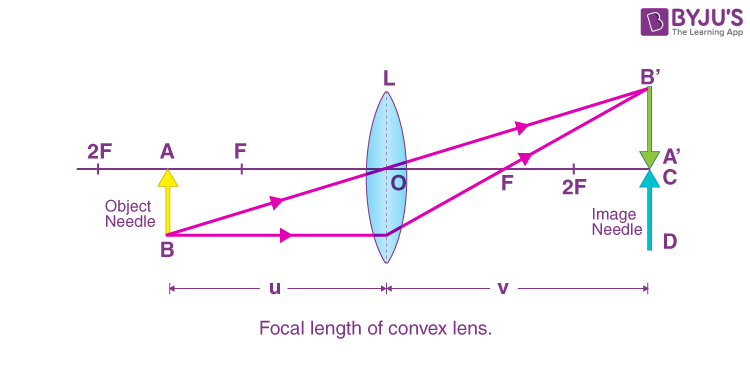## Procedure

1. Fix the convex lens in the holder.
2. Use the lens to focus an object like a tree on a white screen or a wall.
3. Precisely focus the image of the object on the wall or screen until a sharp image is obtained.
4. Calculate the distance between the lens and the wall or screen. It will be the rough focal length of the lens.
5. Mount the holder with the lens in a locked upright, and place the upright at the 50cm mark.
6. Tune the orientation of the lens in such a way that its surface is perpendicular and vertical to the optical bench’s length.
7. Maintain the upright fixed in the above position throughout.
8. Use the thin optical needle as the object (O). Fix it in the outer, laterally movable upright close to zero ends.
9. Adjust the object needle upright, and place it at a distance close to 1.5 times the acquired approximate focal length of the lens.
10. Tune the height of the object needle to adjust its tip to lie on the horizontal plane through the lens’s optical centre. Record the position of the index mark on the foot of the object needle upright.
11. Close your right eye, and open the left eye. Then watch from the other terminal of the optical bench. The image’s tip should be present in the middle of the lens.
12. Place the thick optical image needle in the fourth upright close to the other terminal of the optical bench.
13. Tune the height of the image needle in a way that its tip is visible in line with the image’s tip when seen with the left eye open.
14. Look towards the right. The tips will be separated. The image needle tip and the image tip have parallax. Clear the parallax tip to tip.
15. Record the position of the index mark on the foot of the image needle upright.
16. Note down the position of the index mark on the foot of the upright of the lens, the image needle, and the object needle in the second observation column.
17. Calculate the index correction for length between the tip of the object needle and the optical centre of the lens. Next, find the distance between the tip of the image needle and the optical centre of the lens, as mentioned.

18. Adjust the object needle upright towards the mirror in steps 1cm to obtain observations 1 and 2.
Repeat the process.
19. Adjust the object needle upright away from the mirror in steps of 1cm to obtain observations 6, 5, and 4.
20. Note down all the observations as shown ahead.

## Observations

The rough or approximate focal length of the convex lens = …….. cm
The real length of the index needle x = …….. cm
The visible distance between the lens and the object needle when the index needle is positioned between them, y = …….. cm
The observed distance between the lens and the image needle when the index needle is positioned between them, z = …….. cm
Corrected distance for the object distance ‘u’, x-y = …. cm
Corrected distance for the image distance ‘v’, x-z = …. cm

Table for u, v; 1/u and 1/v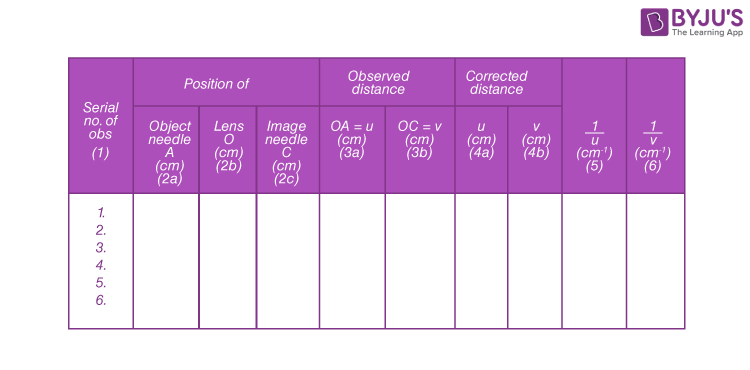## Calculation

Calculation of the focal length by the graphical method:
1) u-v Graph
Choose a suitable but the same scale to denote v along the Y-axis and u along X-axis. As per sign conventions, v is positive, and u is negative. Plot the points for different rates of v and u from the observation table in the second section. The graph is a rectangular hyperbola, as represented in the graph between u and v.

Draw a line OA creating an angle of 45° with either axis bisecting ∠YOX’ and reaching the curve at point A. Draw AC and AB perpendicular on the Y and X’-axis, respectively. The values of v and u are the same for point A. The coordinates of point A should be (2f, 2f), as for a convex lens, when v=2f, u=2f.

Therefore, AB = AC = 2f or OB=OC = 2f

This implies that f = OB/2 and f = OC/2

Mean value of f = …..cm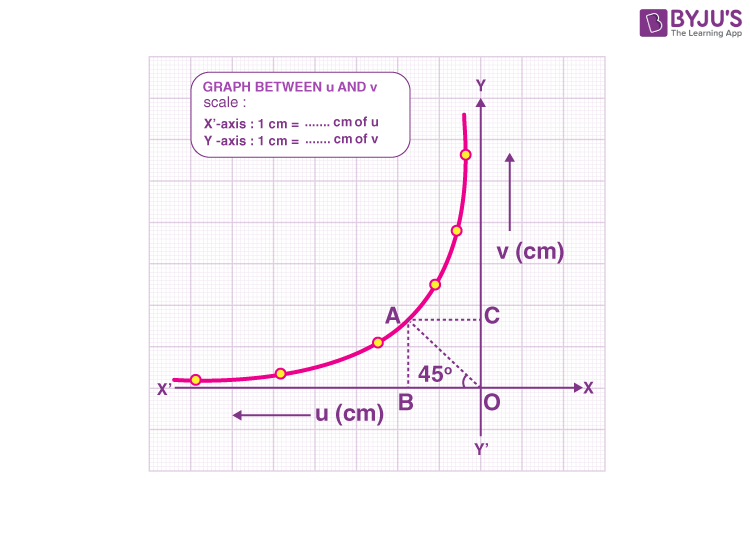2) Choose an apt but the same scale to denote 1/u along the X’-axis and 1/v along the Y-axis. As per sign conventions, 1/u is negative, and 1/v is positive. Plot the points for varied sets of values of 1/u and 1/v from the observation table. The graph evolves to be a straight line as represented in the graph between 1/v and 1/u.

The straight-line slices the two-axis OY and OX’ at an angle of 45° at points Q and P, respectively, creating equal intercepts on the axis. Calculate the distance between OQ and OP.

Then the focal length, f = 1/OP = 1/ OQ

## Explanation

Another u-v Graph (same as a concave mirror)

Choose an apt but the same scale to denote v along the Y-axis and u along the X’-axis. Note the points at distances v1, v2, v3,……. etc., along the OY-axis for various sets of observations from the resulting table.

Create straight lines connecting u3 with u1 with v1, u2 with v2, u3 with v3,………. etc. The lines meet at the intersection point K, as represented in the graph below.

Draw KM and KL perpendicular on the Y-axis and X’-axis, respectively.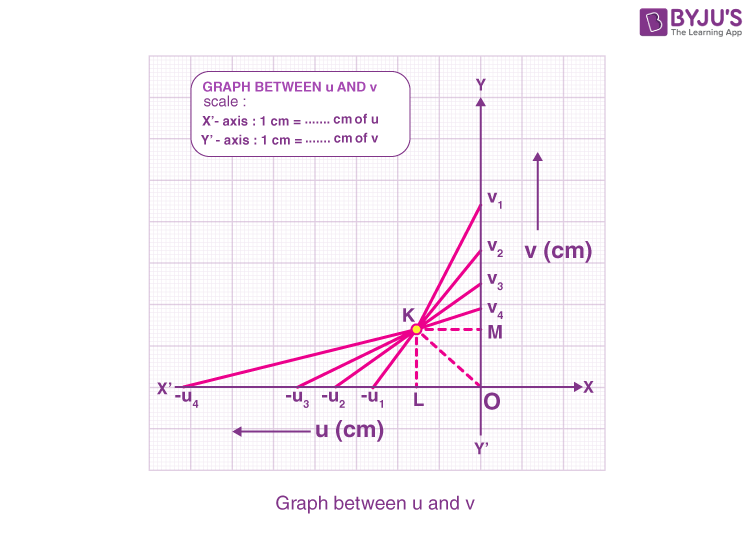F = OL = OM
= …….. cm.

## Result

The focal length of the convex as calculated from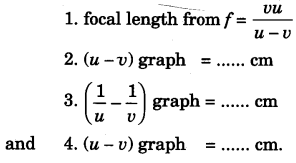## Precautions

a) Tips of the image needle and the object needle must lie at the exact height as the lens’s centre.
b) Parallax must be corrected from tip to tip by placing the eye at a distance minimum of 30 cm apart from the needle.
c) The object needle must be positioned at such a distance that only an inverted and real image of the object needle is produced.
4) Index correction for u and v must be applied.

## Viva Questions

1) What is meant by focal length?
Focal length is the length from a mirror or a lens to the given focal point.

2) What is meant by a convex lens?
It is an optical lens that usually consists of two spherical surfaces. If the lens is bent outwards, the lens is known as a convex lens.

3) What is meant by a concave lens?
A concave lens is an optical lens that usually consists of two spherical surfaces. If the lens is bent inwards, the lens is known as a concave lens.

4) What is meant by an optical centre?
The optical centre is generally stated as the central point of the given lens, along which a light ray passes without experiencing any deviation.

5) What is the general formula for finding the focal length?

$$\begin{array}{l}\frac{1}{f}= \frac{1}{v} – \frac{1}{u}\end{array}$$

This experiment is one of the important experiments in Class 12 Physics Practicals.

Stay tuned to BYJU’S and Fall in Love with Learning!